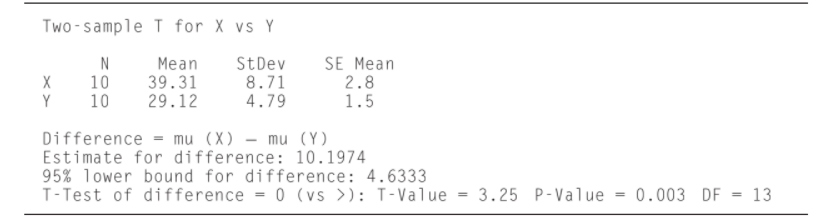×
Get Full Access to Statistics For Engineers And Scientists - 4 Edition - Chapter 6.7 - Problem 16e
Get Full Access to Statistics For Engineers And Scientists - 4 Edition - Chapter 6.7 - Problem 16e

×

# The following MINITAB output presents the | Ch 6.7 - 16EISBN: 9780073401331 38

## Solution for problem 16E Chapter 6.7

Statistics for Engineers and Scientists | 4th Edition

• Textbook Solutions
• 2901 Step-by-step solutions solved by professors and subject experts
• Get 24/7 help from StudySoup virtual teaching assistantsStatistics for Engineers and Scientists | 4th Edition

4 5 1 269 Reviews
22
3
Problem 16E

he following MINITAB output presents the results of a hypothesis test for the difference $$\mu_X-\mu_Y$$ between two population means.a. Is this a one-tailed or two-tailed test?

b. What is the null hypothesis?

c. Can $$H_0$$ be rejected at the 1% level? How can you tell?

Equation Transcription:Text Transcription:

mu_X-mu_Y

H_0

Step-by-Step Solution:
Step 1 of 3

Math 1310: College Algebra Course Syllabus Section number: This information applies to ALL face­to­face sections Delivery format: face­to­face lecture Prerequisites: MATH 1300: Fundamentals of Mathematics or a passing score on the test for placement into College Algebra. Textbook: Available in electronic form (PDF) through CASA for all enrolled students. The information contained in this class outline is an abbreviated description of the course. Additional important information is contained in the departmental policies statement at http://www.math.uh.edu/~dog/13xxPolicies.doc and at your instructor’s personal webpage. You are responsible for knowing all of this information. Upon successful completion of this course, students will be able to apply algebraic rules and transformations to simplify or elaborate on mathematical expressions. Students will understand and be able to apply methods of solution of polynomial equations and will understand the properties of roots of such equations. They will be able to solve systems of linear equations and inequalities. They will understand properties of exponential and logarithmic functions and will be able to solve equations involving these functions. Students will be able to use graphical information and symbolic expression simultaneously in solving mathematical problems. They will be able to translate ordinary language descriptions of a problem into mathematical expression, i.e., they will be able to solve stated problems usin

Step 2 of 3

Step 3 of 3

## Discover and learn what students are asking

Statistics: Informed Decisions Using Data : Tests for Independence and the Homogeneity of Proportions
?Celebrex Celebrex, a drug manufactured by Pfizer, Inc., is used to relieve symptoms associated with osteoarthritis and rheumatoid arthritis in adults.

Unlock Textbook Solution